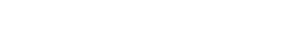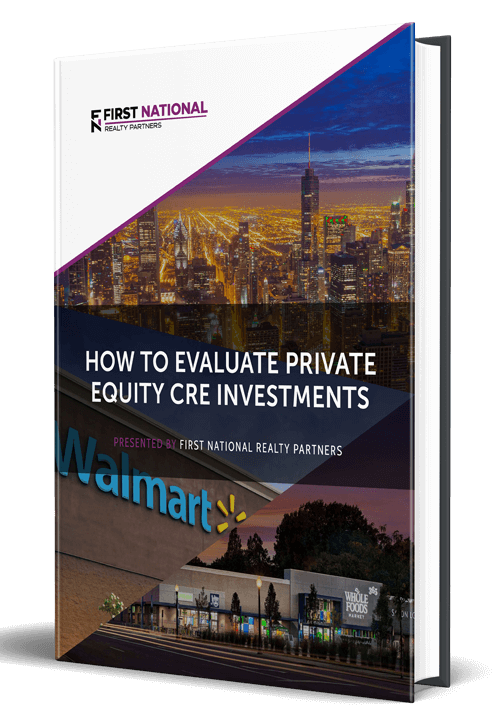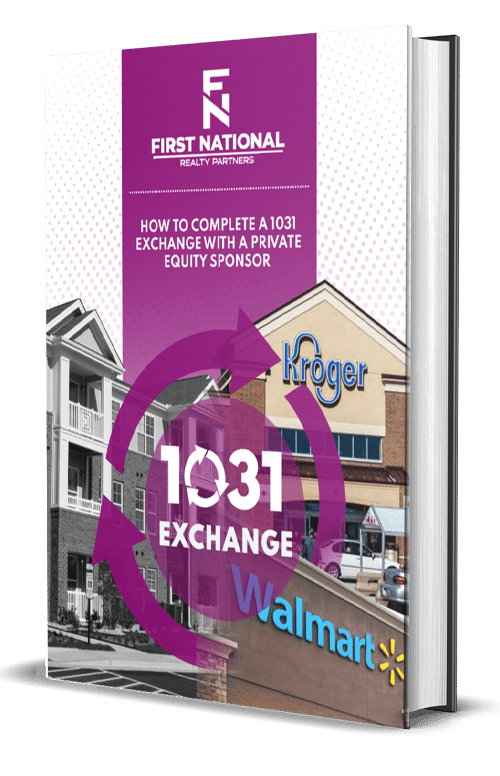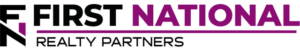# Cap Rate vs Cash on Cash Return: What is the Difference?

### Key Takeaways

• A property’s cap rate is a performance metric that represents the relationship between net operating income and value.
• The cap rate calculation formula is NOI divided by purchase price.  However, these numbers are not always known at the time the cap rate is being calculated.  But, as long as two of the three variables are known, the missing one can be solved for.
• Cash on cash return represents a commercial real estate investor’s annual return on total cash invested.
• The cash on cash return formula is annual cash received divided by total cash invested.
• There are two CoC varieties, the “unlevered” cash on cash return, which does not take into account the impact of debt, and the “levered” cash flow which does.
• ​Neither metric is “better” than the other, but they are useful at different stages of the transaction lifecycle.  The cap rate is most useful as an initial screen for deals that do not meet a basic set of criteria.  The cash on cash return is to be used later, when more detailed analysis is performed.

For individual real estate investors, one of the most important considerations is an investment’s potential profitability.  However, estimating potential profit can be a confusing and inexact process because there are multiple metrics used to measure it and each of them are used for a different purpose and require their own inputs.

In this article, we will focus on two of the most important return metrics – the Cap Rate and the Cash on Cash Return – by describing how they are used, how to calculate them, why they are important, and the pros and cons of using each as a tool for estimating potential profitability.  By the end of the article, readers will know how to calculate both return metrics and identify what drives changes in each of them.

At First National Realty Partners, we always use the Cap Rate and the Cash on Cash Return when underwriting the potential acquisition of an investment property.  To learn more about our current investment opportunities, click here.

## The Cap Rate Explained

The capitalization rate (cap rate) is a widely used and very versatile investment return metric.  Yet, it can also be very misunderstood.

The easiest way to think about the cap rate is that it highlights the relationship between a property’s value and its net operating income (NOI).  The formula used to calculate it is:

Cap Rate = Net Operating Income / ( Purchase Price / Value )

To understand why this is a versatile and occasionally confusing metric, it is helpful to break the above equation into its components.

A property’s Net Operating Income is calculated as all sources of income less operating expenses.  For example, if a property had \$100 in total income and \$50 in operating expenses, the resulting NOI would be \$50.

In the denominator, there are multiple options that could be used to represent a property’s value.  In a purchase transaction, it could be the purchase price or appraised value.  Or, in a non-purchase, it could just be an estimate based on comparable sales.

Now, the confusing part is that these inputs aren’t always known when trying to calculate the cap rate.  But, if a commercial real estate investor has 2 of the three (cap rate, NOI, property value), the missing one can be calculated.  This point can be illustrated by highlighting a common scenario in commercial real estate pre-purchase due diligence.

Suppose that a real estate investor is interested in purchasing a particular commercial rental property, but they aren’t sure what price to offer.  To estimate it, they could obtain the property’s NOI from historical operating statements and the cap rate from recent sales of similar properties in the same market.  With these two variables, a reasonable purchase price can be calculated by dividing NOI by the cap rate.

### Advantages Of The Cap Rate

​There are three key advantages to using the cap rate as a measure of return on a real estate investment.

The first is the most obvious.  It is easy to calculate and the inputs needed are either readily available or can be estimated with ease.  This also allows for a simple way to compare potential returns across property types and asset classes.

Second, the cap rate provides some insight into the potential return on a property.  In fact, the resulting percentage represents what a real estate investor could earn if they purchased the property with cash.  If debt is used in the purchase, the return would be higher than the cap rate.

Third, because there is an inverse relationship between risk and return, the cap rate is also a quick way to gauge the market’s assessment of risk in a property.  A higher cap rate represents more risk, which means that the property’s purchase price is lower.  Conversely, a lower cap rate represents less risk, so the value of the property is higher.  To put this point in perspective, a “low” cap rate would be in the 4% – 6% range and a “high” cap rate would be above 10%.  But, it is important to remember that cap rates are unique to each deal.

Because the cap rate represents an annual return on investment, it is often compared to another return metric known as the cash on cash return (CoC return).

## Cash on Cash Return Explained

Remember, the cap rate represents the annual rate of return on a potential investment, assuming it was purchased with all cash.  But, commercial real estate assets are rarely purchased with cash.  A typical transaction includes a combination of a cash down payment and some loan proceeds.

With this in mind, the cash on cash return represents a real estate investor’s annual return on the total cash invested and there are two varieties.  The “unlevered” cash on cash return represents the return with no debt in the transaction and it could be close to the cap rate.  But, the “levered” cash on cash return will be higher because it includes the impact of debt.  The formula used to calculate cash on cash return is:

Cash on Cash Return = Cash Received In a Given Year / Total Cash Invested

For example, if a real estate investor receives \$10,000 in a year on a \$100,000 investment, their cash on cash return is 10%.

So, the key difference between the cap rate and the levered cash on cash return is that the CoC return includes the impact of debt in the transaction.

### Advantages of The Cash on Cash Return

Like the cap rate, the cash on cash return is also easy to calculate with widely available inputs.  But, the primary benefit of using this metric is that it provides a more realistic picture of the property’s financial performance because it accounts for the impact of the leverage used in the transaction.

For properties that carry higher risk, it would be expected that they would also come with a higher cash on cash return.  Likewise, a lower cash on cash return would be expected for a property that has less risk.

## When to Use Each Metric

When comparing the cap rate to the cash on cash return, neither one is an objectively “better” way of measuring real estate investment returns.  They are just different and they have different utility in different circumstances.  To be clear, they should both be used when evaluating a potential real estate investment opportunity, just at different times.

### When to Use The Cap Rate

The cap rate can be a helpful way to screen deals.  For example, if a commercial real estate investor requires a cap rate of 8% or higher, they could quickly screen out any deal with a cap rate below that.  This type of screen can prevent real estate investors from wasting time evaluating deals that don’t meet their return criteria.

### When to Use The Cash on Cash Return

For the remaining deals, the cash on cash return is a more detailed measurement of potential profitability.  While a “good” return is different for every investor, 8% to 15% levered cash on cash return is usually considered acceptable.  But, this is also measured relative to the “risk free rate”, which is the interest rate paid on a 10-year US Treasury bill.  As such, a real estate investor may not necessarily be looking for an objective return, but a spread over the risk free rate.

Cap rate and cash on cash return are not the only return metrics used to measure real estate investing profitability.  Other metrics include:

• Internal Rate of Return (IRR):  The IRR is the rate of return on each dollar invested, for each period of time it is invested in.
• Equity Multiple:  The equity multiple is a measure of the total cash received from an investment divided by the total cash investment.
• Debt Service Coverage Ratio:  The DSCR is a measure of a property’s net operating income divided by the total annual debt service.  In other words, it is a measure of the relationship between a property’s annual cash flow to the annual financing costs.

## Summary and Conclusions

Commercial real estate cap rate is a performance metric that represents the relationship between net operating income and value.  The cap rate calculation formula is NOI divided by purchase price.  However, these numbers are not always known at the time the cap rate is being calculated.  But, as long as two of the three variables are known, the missing one can be solved for.

Cash on cash return represents a commercial real estate investor’s annual return on total cash invested.  The cash on cash return formula is annual cash received divided by total cash invested.  There are two CoC varieties: “unlevered” and “levered” cash flow. The “unlevered” cash on cash return does not take into account the impact of debt, and the “levered” cash flow does.

​Neither metric is “better” than the other, but they are useful at different stages of the transaction lifecycle.  The cap rate is most useful as an initial screen for deals that do not meet a basic set of criteria.  The cash on cash return is to be used later, when more detailed analysis is performed.

## Interested In Learning More?

First National Realty Partners is one of the country’s leading private equity commercial real estate investment firms. With an intentional focus on finding world-class, multi-tenanted assets well below intrinsic value, we seek to create superior long-term, risk-adjusted returns for our investors while creating strong economic assets for the communities we invest in.

Get instant access to all of our current and past commercial real estate deals.## Subscribe Now

Get the latest news on real estate

FNRP Spotlight Series

1031 Exchange

Acquisitions

## Get More From FNRP## How to Evaluate Private Equity CRE Investments## How to Complete a 1031 Exchange with a Private Equity Sponsor